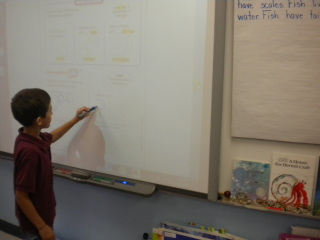Sort 2D Shapes

8 teachers like this lesson
Print Lesson

Objective

SWBAT use defining attributes to sort shapes.

Big Idea

In this lesson, students will be able to reason with shapes and sort them by their defining attributes.

Activating Strategy

10 minutes

I begin this lesson by reading the book “A Circle Here, A Square There”.  I like to use this book because each picture shows the shapes in everyday objects.

Review the attributes of triangles and rectangles. Remind children of the terms vertex and side to describe two-dimensional shapes. On the board, draw a triangle and an open three-sided shape.  Ask the students:

• Which is a triangle? Why? (Children should identify the triangle and explain that it has 3 sides and 3 vertices, or corners, and is closed.)

Tell children that the open shape is not a triangle because although it does have 3 sides, a triangle should be closed. Repeat the activity with a rectangle and an open four-sided shape.  One common student misconception of 1.G.A.1 that I anticipate is that students may focus on the orientation of the shape instead of its relative position or attributes.  In this lesson about two-dimensional shapes, children distinguish between defining attributes, such as number of sides and being closed or open, and non-defining attributes, such as color, orientation, and overall size. They learn that both common and different looking two-dimensional shapes, also known as flat and plane shapes, can be sorted in more than one way.

Teaching Strategies

15 minutes

I display the first slide of the Sort 2D shapes.ppt and read the following problem aloud to the class:

Devon wants to sort these shapes to show a group of triangles and a group of rectangles.

I point out that these are two-dimensional shapes, but they are also known as flat shapes or plane shapes.  I want students to know and use these terms fluidly.

Then I initiate and guide a discussion by asking the following of the group:

• How are the shapes alike and different? (number of sides/vertices, color, size, open or closed)
• How can you sort these shapes into two groups? (rectangles/triangles, red/blue, 3 sides/4 sides, open/closed, big/small)I like to do turn and talks and then whole group share outs for the discussion, and, by the end, I want to make sure children understand that the shapes on the slide need to be sorted into two groups as triangles and rectangles, regardless of their orientation.

Here are additional questions that I ask to surface this understanding:

• Are there some shapes that are not triangles or rectangles? How do you know? (Yes. The open shapes are not triangles or rectangles because their sides are not closed.)
• Can you sort by color to show a group of triangles and a group of rectangles? Explain. (No. Rectangles and triangles would be in both groups.)
• Can you sort by size to show a group of triangles and a group of rectangles? Explain.  (No. Rectangles and triangles would be in both groups.)
• How can you sort a group of triangles and a group of rectangles? (by the shape)Then I work through the model on the top of the worksheet with children. I guide them to see that the sorting rules describe ways the shapes are alike and different. I want to make sure children understand that a square is a special kind of rectangle because it has four sides that are all the same length.  Making sure that students develop an understanding of the pattern and structure of 2D shapes (MP7) is important for building their foundational understanding of geometry.  They need to understand that there is a pattern and structure in geometrical shapes in order to sort them by their properties.

• What do you notice about all of the shapes in the first group? (They are all curved and closed.)
• To be a triangle, does the size define the shape? Does the color define the shape? Explain. (No. A triangle can be any size or color, but it always has 3 sides and 3 vertices.)

Independent Practice

30 minutes

For the independent practiced portion of this lesson, I hand out the Sort 2D Shapes_worksheet.docx.  We talk through the model on the top of the page and do the first few problems together as a whole group.  Once most students have the concept, I turn them loose to complete the worksheet independently.

This is a sample of high quality student work:

Closing/Summarizing

5 minutes

To close out this lesson, I have students draw a square and a rectangle in their journal and explain a sorting rule for both shapes.